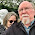## Monday, July 7, 2014

### #70Days A few good problems for systems of equations

32/70

The other day I wrote about a compare contrast activity involving two systems of equations problems.  Today I want to mention some of the other problems I plan for that unit.  Systems of equations is our second unit - right after introducing foundations of functions.  We don't spend any real class time on linear functions since they are covered so fully in Algebra 1.  Instead we review significant thoughts about linear functions as we solve the systems.

In our systems unit these are some of the problems I want us to discuss:

Tortoise, Hare and Rat from The Math Projects Journal: In this problem students have to work through units of measurement as well as solving the system.

The Cash Box from Illustrative Mathematics: I like this because students have to decide if the dollar on the floor should be in the cash box.

Going to the Game from Yummy Math: These are basic problems and could be used as a pre-test to see what students remember from previous studies.

Flicks and Datelines from Mathalicious: These require a subscription.  Datelines may have teen appeal more so than other problems!

We will also do an OREOS problem but I will save that one for the unit on matrices!

Fawn Nguyen has a fun Legos problem as well ... for optimization! She also describes showing students how to use excel to solve problems - great idea if you have technology available!

Check out Enriching Mathematics for all sorts of systems problems. These are not your typical textbook problems! I especially like the problem called "Intersections."  It gives 2 sets of simultaneous equations and asks: Explain why the solutions are so different and yet the pairs of equations are nearly identical.  Can't wait to listen in on these discussions!  I may ask students to record their conversations!

Most of the above are not typical textbook problems.  Do you have suggestions for systems problems that are essential for students to encounter??

#### 1 comment:

1.I should have checked the Enriching Math site ... awesome set of puzzling systems problems there! Not "word" problems per se but fun anyway! http://nrich.maths.org/public/leg.php?code=53